Open in App
Not now

# Difference between loc() and iloc() in Pandas DataFrame

• Difficulty Level : Easy
• Last Updated : 08 Aug, 2022

Pandas library of python is very useful for the manipulation of mathematical data and is widely used in the field of machine learning. It comprises many methods for its proper functioning. loc() and iloc() are one of those methods. These are used in slicing data from the Pandas DataFrame. They help in the convenient selection of data from the DataFrame in Python. They are used in filtering the data according to some conditions.

### Creating a sample dataframe

The working of both of these methods is explained in the sample dataset of cars.

## python3

 `# importing the module` `import` `pandas as pd`   `# creating a sample dataframe` `data ``=` `pd.DataFrame({``'Brand'``: [``'Maruti'``, ``'Hyundai'``, ``'Tata'``,` `                               ``'Mahindra'``, ``'Maruti'``, ``'Hyundai'``,` `                               ``'Renault'``, ``'Tata'``, ``'Maruti'``],` `                     ``'Year'``: [``2012``, ``2014``, ``2011``, ``2015``, ``2012``,` `                              ``2016``, ``2014``, ``2018``, ``2019``],` `                     ``'Kms Driven'``: [``50000``, ``30000``, ``60000``,` `                                    ``25000``, ``10000``, ``46000``,` `                                    ``31000``, ``15000``, ``12000``],` `                     ``'City'``: [``'Gurgaon'``, ``'Delhi'``, ``'Mumbai'``,` `                              ``'Delhi'``, ``'Mumbai'``, ``'Delhi'``,` `                              ``'Mumbai'``, ``'Chennai'``,  ``'Ghaziabad'``],` `                     ``'Mileage'``:  [``28``, ``27``, ``25``, ``26``, ``28``,` `                                  ``29``, ``24``, ``21``, ``24``]})`   `# displaying the DataFrame` `display(data)`

Output :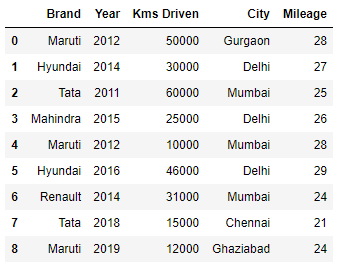## Python loc() function

The loc() function is label based data selecting method which means that we have to pass the name of the row or column which we want to select. This method includes the last element of the range passed in it, unlike iloc(). loc() can accept the boolean data unlike iloc(). Many operations can be performed using the loc() method like

### Example 1:

Selecting data according to some conditions

## python3

 `# selecting cars with brand 'Maruti' and Mileage > 25` `display(data.loc[(data.Brand ``=``=` `'Maruti'``) & amp` `                 ``(data.Mileage & gt` `                  ``25``)])`

Output :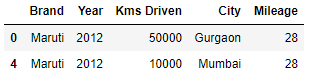### Example 2:

Selecting a range of rows from the DataFrame

## python3

 `# selecting range of rows from 2 to 5` `display(data.loc[``2``: ``5``])`

Output :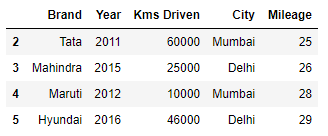### Example 3:

Updating the value of any column

## python3

 `# updating values of Mileage if Year < 2015` `data.loc[(data.Year & lt` `          ``2015``), [``'Mileage'``]] ``=` `22` `display(data)`

Output :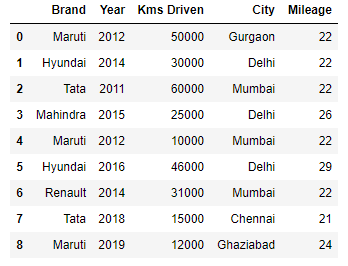## Python iloc() function

The iloc() function is an indexed-based selecting method which means that we have to pass an integer index in the method to select a specific row/column. This method does not include the last element of the range passed in it unlike loc(). iloc() does not accept the boolean data unlike loc(). Operations performed using iloc() are:

### Example 1:

Selecting rows using integer indices

## python3

 `# selecting 0th, 2th, 4th, and 7th index rows` `display(data.iloc[[``0``, ``2``, ``4``, ``7``]])`

Output :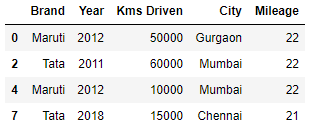### Example 2:

Selecting a range of columns and rows simultaneously

## python3

 `# selecting rows from 1 to 4 and columns from 2 to 4` `display(data.iloc[``1``: ``5``, ``2``: ``5``])`

Output :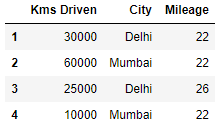My Personal Notes arrow_drop_up
Related Articles